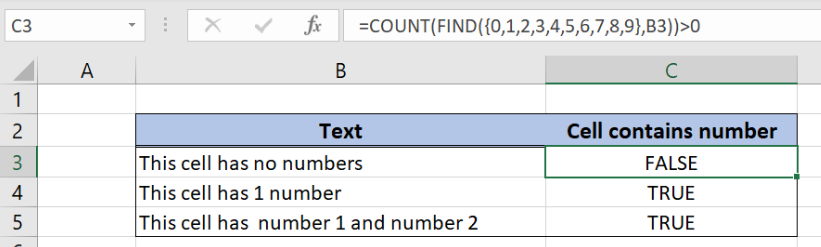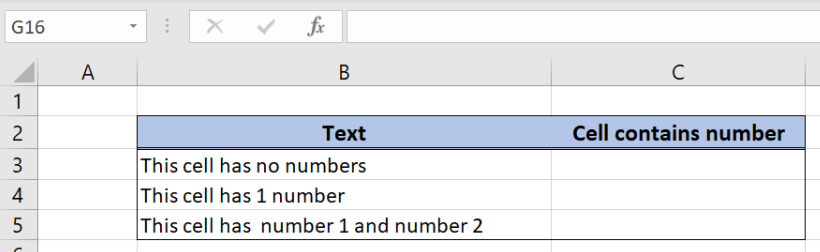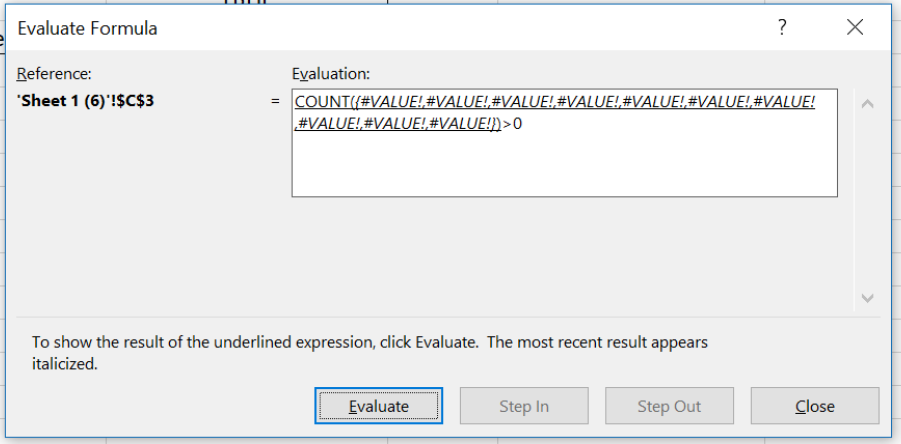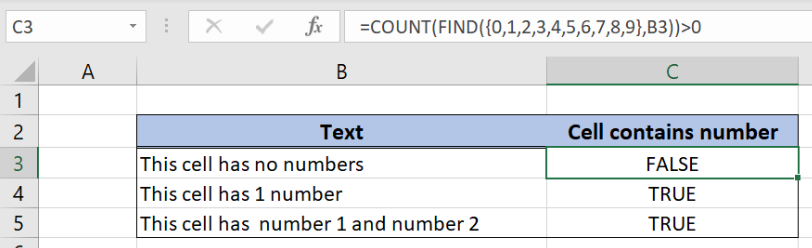Get instant live expert help with Excel or Google Sheets“My Excelchat expert helped me in less than 20 minutes, saving me what would have been 5 hours of work!”

#### Post your problem and you’ll get Expert help in seconds.

Your message must be at least 40 characters
Our professional Expert are available now. Your privacy is guaranteed.

# Cell contains number

Excel allows a user to check if a cell contains a number by using the COUNT and FIND functions. This step by step tutorial will assist all levels of Excel users in checking if a cell contains any number.Figure 1. The result of the COUNT and FIND functions

## Syntax of the FIND Formula

The generic formula for the FIND function is:

`=FIND(find_text, within_text)`

The parameters of the FIND function are:

• find_text – a text that we want to find in selected text or cell
• within_text – a text or cell where we want to find a text.

If a text is found, the FIND function returns a position of text in a cell. If it’s not found, the function returns #VALUE! error.

## Syntax of the COUNT Formula

The generic formula for the COUNT function is:

`=COUNT(value1, value2, ...., valueN)`

The parameters of the COUNT function are:

• value1, value2, …, valueN – values that we want to count.

## Setting up Our Data for the COUNT FunctionFigure 2. Data that we will use in the COUNT example

Let’s look at the structure of the data we will use. In column B we have texts, while in column C we want to return TRUE if the cell contains number and FALSE if not.

## Check if cells contain a number

In our example, we want to check if texts from column B contain any number and return a result in column C.

The formula looks like:

`=COUNT(FIND({0,1,2,3,4,5,6,7,8,9}, B3)) > 0`

The parameter find_text of the FIND function is the array of numbers {0,1,2,3,4,5,6,7,8,9}. The within_text parameter is B3. When using the array, the FIND function will return a result for every element in the array. Let’s evaluate the formula and see the result:Figure 3. Evaluate the FIND function

The FIND function returned #VALUE! error for every element of the array, as there is no number in the array.

All of the results of the FIND function are parameters for COUNT function. As errors are not counted, the function returns 0. In the end, we check if the result of the COUNT is greater than 0. As it’s not, the result in the cell C3 is FALSE.

To apply the COUNT function, we need to follow these steps:

• Select cell C3 and click on it
• Insert the formula: `=COUNT(FIND({0,1,2,3,4,5,6,7,8,9}, B3)) > 0`
• Press enterFigure 4. Using the COUNT and FIND functions to check if cells contain a number

Most of the time, the problem you will need to solve will be more complex than a simple application of a formula or function. If you want to save hours of research and frustration, try our live Excelchat service! Our Excel Experts are available 24/7 to answer any Excel question you may have. We guarantee a connection within 30 seconds and a customized solution within 20 minutes.

### Did this post not answer your question? Get a solution from connecting with the expert.Another blog reader asked this question today on Excelchat:
Solution examplesI need to count number of dates from a timestamped date in column from the completed date column to show the number of days. I have not successfully been able to do this. I am a novice Excel user. Can you help?
Solved by O. B. in 15 minsUse the Vlookup Function to complete the "employee" column of table 2. Use "job Id" from table 2 as your lookup_value(s) and table 1 as your reference.
Solved by C. H. in 16 minsIf a cell in another sheet is populated I need a vlookup done. If the cell is not populated I need the cell to return blank.
Solved by T. D. in 60 minsI am trying to make a chart that turns a week range red if nothing is entered in the range. If something is entered then I would like it to turn green. Please Help
Solved by E. U. in 43 minsI need a check box to show/hide an answer of an if function
Solved by Z. U. in 23 mins## Subscribe to Excelchat.coAnother blog reader asked this question today on Excelchat: